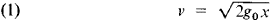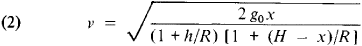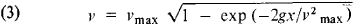# Fall of a Body

The following article is from The Great Soviet Encyclopedia (1979). It might be outdated or ideologically biased.

## Fall of a Body

the motion of a body in the earth’s gravitational field when the body’s initial velocity is equal to zero. The fall of a body occurs under the action of gravitational attraction, which is dependent on the distance r to the center of the earth. The body’s fall is also affected by the resistance of the medium —air or water—through which the body travels; this resistance is dependent on the body’s velocity v. Another factor influencing the fall of a body with respect to the surface of the earth is the earth’s diurnal rotation with an angular velocity ω ≈ 0.0000729 radians/sec.

For bodies that fall from a very low height (the rate of fall is low) or from a very great height (most of the path is in a vacuum), air resistance can be essentially ignored. If we also ignore the earth’s rotation, because ω is small, and the earth’s lack of perfect sphericity, the motion of the center of gravity of a falling body will occur along a straight line directed toward the center of the earth. For the fall of a body from a height h—where h is the distance from the earth’s surface—that is very small in comparison with the earth’s radius R, the dependence of the gravitational force on r can be disregarded; thus the body’s center of gravity can be assumed to move with a constant gravitational acceleration g0 and with a velocity that increases according to the lawwhere x is the distance traveled from the initial position. When h is large, the dependence of the gravitational force on the distance r = R + hx must be taken into account. The acceleration of the center of gravity of a falling body varies according to the law w = g0R2r2, and the velocity isWhen x = h, formula (2) gives the velocity at the instant of impact with the earth; when hR, the formula becomes formula (1).

The basic influence of the earth’s rotation on the fall of a body from a low height is taken into account by adding the vehicle (centrifugal) force to the force of gravitation. The sum of these two forces gives the force of gravity P, which is directed along the vertical and under whose action the body falls. The free fall acceleration, or the acceleration of the force of gravity, g differs somewhat from go both numerically and in direction and depends on the geographical latitude ϕ. The magnitude of the velocity is also determined by formula (1), with g0 replaced by g. In a first approximation, the Coriolis force, which is an additional effect of the earth’s rotation, causes the deflection of falling bodies from the vertical toward the east.

If the resistance of the medium is taken into account, the law governing the fall of a body is considerably changed. When the velocity of fall does not reach the sound velocity (in air this is essentially the case when v < 300 m/sec), the force of resistance is F – 0.5cx pSV2, where S is the area of the maximum cross section, ρ is the air density, and cx is the coefficient of resistance, which depends on the shape of the body. In this law of resistance the velocity of fall is, if ρ and g are assumed to be constant,where vmax = 2P/(cxρS). It can be seen from formula (3) that with increasing x the velocity v tends toward vmaz, which is the limiting velocity of fall. When cx and S are sufficiently great, the value of v becomes close to vmax over a small initial segment of the path and the subsequent fall of the body occurs with a practically constant velocity vmax.

S. M. TARG